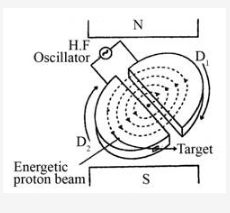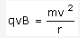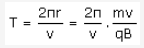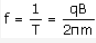# Draw a schematic diagram of a cyclotron. Explain its underlying principle and working

Draw a schematic diagram of a cyclotron. Explain its underlying principle and working, stating clearly the function of the electric and magnetic fields applied on a charged particle. Deduce an expression for the period of revolution and shown that it does not depend on the speed of the charged particle.

Cyclotron: Cyclotron is a device by which the positively charged particles like protons, deutrons, etc. can be accelerated.

Principle: Cyclotron works on the principle that a positively charged particle can be accelerated by making it to cross the same electric field repeatedly with the help of a magnetic field.Construction: The construction of a simple cyclotron is shown in figure above. It consists of two-semi-cylindrical boxes D1 and D2, which are called dees. They are enclosed in an evacuated chamber. The chamber is kept between the poles of a powerful magnet so that uniform magnetic field acts perpendicular to the plane of the dees. An alternating voltage is applied in the gap between the two dees by the help of a high frequency oscillator. The electric field is zero inside the dees Working and theory: At a certain instant, let D1 be positive and D2 be negative. A proton from an ion source will be accelerated towards D2, it describes a semi-circular path with a constant speed and is acted upon only by the magnetic field. The radius of the circular path is given by,From the above equation we get,------ (I)
The period of revolution is given by,(From I)The frequency of revolution is given by,From the above equation it follows that frequency f is independent of both v and r and is called cyclotron frequency. Also if we make the frequency of applied a.c. equal to f, then every time the proton reaches the gap between the dees, the direction of electric field is reversed and proton receives a push and finally it gains very high kinetic energy. The proton follows a spiral path and finally gets directed towards the target and comes out from it.